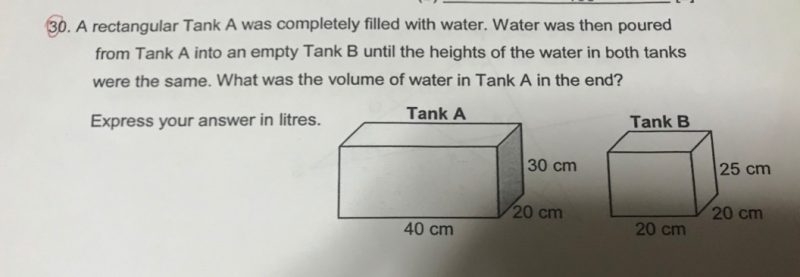# QuestionHi All,

Thanks!

Initial volume of water in Tank A = (40 x 30 x 20) cm3 = 24,000 cm3

Area of base of Tank A = (40 x 20) cm2 = 800 cm2

Area of base of Tank B = (20 x 20) cm2 = 400 cm2

After pouring, let h be the height of water in both tanks

Volume of water in Tank A = (800h) cm3

Volume of water in Tank B = (400h) cm3

Total volume of water = (800h + 400h) cm3 = 24,000 cm3

1200 h = 24,000

h = 20 cm

Volume of water in Tank A = (800 x 20) cm3 = 16,000 cm3 = 16L

0 Replies 1 Like ✔Accepted Answer

40 x 20 x 30 = 24000 (amount of water in Tank A at first)
24000/[(40 x 20) + (20 x 20)] = 20 (height of water in both tanks after water was then poured from Tank A to Tank B)
20 x 40 x 20 = 16000
16000 cm^3 = 16 l

Ans : 16 l.

0 Replies 0 Likes

This type of question is easy if we know the height in the end.

Because we do not know the height, we make it 1u

40 x 20 x 1u = 800u     (volume of water in Tank A, in terms of “u”)

20 x 20 x 1u = 400u     (volume of water in Tank B, in terms of “u”)

800u + 400u = 1200u (total volume of water, in terms of “u”)

40 x 20 x 30 = 24000 (volume of water at first)

1200u = 24000             (total volume of water)

1u = 20                            (height of water in the end)

40 x 20 x 20 = 16000  (volume of water in Tank A in the end)

Ans: 16 litres

We are using 1u to solve the above question.

Questions can varied, like height of Tank A is twice of Tank B.

Therefore, height of Tank A is 2u and Tank B is 1u.

And we can solve the question using the above concept.

0 Replies 0 Likes